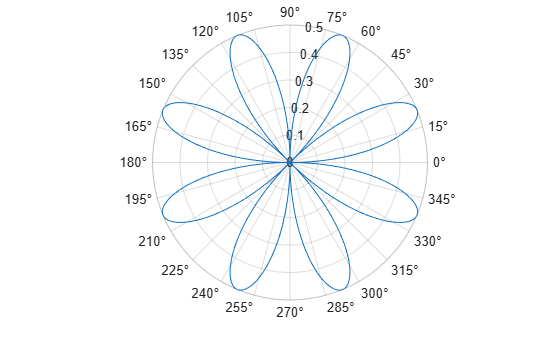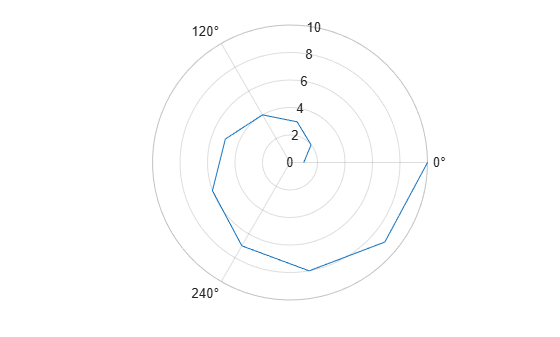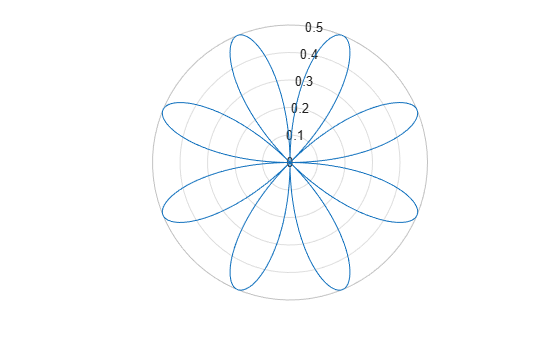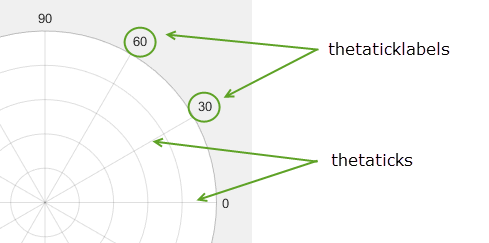thetaticks

Set or query theta-axis tick values

Description

example

thetaticks(ticks) sets the theta-axis tick values, which are the locations along the theta-axis where the tick marks and grid lines appear. Specify ticks as a vector of increasing values; for example, [0 90 180 270]. This command affects the current axes.

tv = thetaticks returns the current theta-axis tick values as a vector.

example

thetaticks('auto') sets an automatic mode, enabling the axes to determine the theta-axis tick values. Use this option if you change the tick values and then want to set them back to the default values.

thetaticks('manual') sets a manual mode, freezing the theta-axis tick values at the current values. Use this option if you want to retain the current tick values when resizing the axes or adding new data to the axes.

m = thetaticks('mode') returns the current theta-axis tick labels mode, which is either 'auto' or 'manual'. By default, the mode is automatic unless you specify tick values or change the mode to manual.

example

___ = thetaticks(pax,___) uses the axes specified by pax instead of the current axes. Specify pax as the first input argument for any of the previous syntaxes.

Examples

collapse all

Create a polar plot. Display tick marks and grid lines along the theta-axis at 0, 90, 180, and 270 degrees. Then, specify a label for each tick mark.

theta = linspace(0,2*pi);
rho = theta/10;
polarplot(theta,rho)

thetaticks([0 90 180 270])
thetaticklabels({'East','North','West','South'})Display tick marks along the theta-axis at nonuniform values between 0 and 360 degrees.

theta = linspace(0,2*pi);
rho = theta/10;
polarplot(theta,rho)
thetaticks([0 30 60 90 180 210 240 270])Display tick marks along the theta-axis every 15 degrees.

theta = 0:0.01:2*pi;
rho = sin(2*theta).*cos(2*theta);
polarplot(theta,rho)
thetaticks(0:15:360)Create a polar plot and specify the theta-axis tick values. Then, set the theta-axis tick values back to the default values.

polarplot(1:10)
thetaticks([0 120 240])thetaticks('auto')Create polar axes and return the polar axes object pax. Ensure that thetaticks affects the polar axes you just created by passing pax as the first input argument to the function.

pax = polaraxes;
thetaticks(pax,0:45:360)Remove the tick marks and grid lines along the theta-axis by specifying the tick values as an empty array.

theta = 0:0.01:2*pi;
rho = sin(2*theta).*cos(2*theta);
polarplot(theta,rho)
thetaticks([])Input Arguments

collapse all

Tick values, specified as a vector of increasing values. The tick values are interpreted in units determined by the ThetaAxisUnits property. By default, the units are degrees.

If you do not want tick marks along the theta-axis, specify an empty vector [].

Example: thetaticks([0 90 180 270])

Example: thetaticks([0:30:330])

Example: thetaticks([])

Note

To specify the tick labels, use the thetaticklabels function.

Polar axes, or an array of polar axes. If you do not specify this argument, then thetaticks modifies the current axes (provided that the current axes is a polar axes object).

Output Arguments

collapse all

Current tick values, returned as a vector.

Current mode, returned as one of these values:

• 'auto' — Axes automatically determines the theta-axis tick values.

• 'manual' — Axes uses manually specified theta-axis tick values.

collapse all

Tick Values

The tick values are the locations along the theta-axis where the tick marks appear. The tick labels are the labels that you see next to each tick mark. Set the tick values using the thetaticks function. Set the corresponding tick labels using the thetaticklabels function.Algorithms

The thetaticks function sets and queries several axes properties related to the theta-axis tick values.

• ThetaTick — Property that stores the theta-axis tick values.

• ThetaTickMode — Property that stores the theta-axis tick value mode. When you set the theta-axis tick values, this property changes to 'manual'.